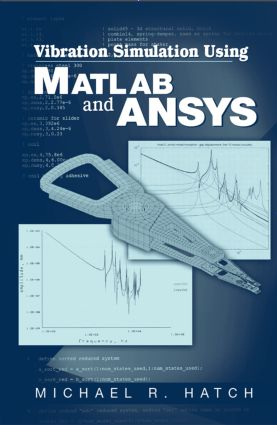# Vibration Simulation Using MATLAB and ANSYS

## 1st Edition

Chapman and Hall/CRC

676 pages

Hardback: 9781584882053
pub: 2000-09-21
\$180.00
x

FREE Standard Shipping!

### Description

Transfer function form, zpk, state space, modal, and state space modal forms. For someone learning dynamics for the first time or for engineers who use the tools infrequently, the options available for constructing and representing dynamic mechanical models can be daunting. It is important to find a way to put them all in perspective and have them available for quick reference.

It is also important to have a strong understanding of modal analysis, from which the total response of a system can be constructed. Finally, it helps to know how to take the results of large dynamic finite element models and build small MATLAB® state space models.

Vibration Simulation Using MATLAB and ANSYS answers all those needs. Using a three degree-of-freedom (DOF) system as a unifying theme, it presents all the methods in one book. Each chapter provides the background theory to support its example, and each chapter contains both a closed form solution to the problem-shown in its entirety-and detailed MATLAB code for solving the problem.

Bridging the gap between introductory vibration courses and the techniques used in actual practice, Vibration Simulation Using MATLAB and ANSYS builds the foundation that allows you to simulate your own real-life problems.

Features

• Demonstrates how to solve real problems, covering the vibration of systems from single DOF to finite element models with thousands of DOF

• Illustrates the differences and similarities between different models by tracking a single example throughout the book

• Includes the complete, closed-form solution and the MATLAB code used to solve each problem

• Shows explicitly how to take the results of a realistic ANSYS finite element model and develop a small MATLAB state-space model

• Provides a solid grounding in how individual modes of vibration combine for overall system response

Introduction

Transfer Function Analysis

Frequency Response Analysis

Zeros in SISO Mechanical Systems

State Space Analysis

State Space: Frequency Response, Time Domain

Modal Analysis

Frequency Response: Modal Form

Transient Response: Modal Form

Modal Analysis: State Space Form

Frequency Response: Modal State Space Form

Time Domain, Modal State Space Form

Finite Elements: Stiffness Matrices

Finite Elements: Dynamics

SISO State Space MATLAB Model from ANSYS Model

Ground Acceleration MATLAB Model from ANSYS Model

SISO Disk Drive Actuator Model

Balanced Reduction

MIMO Two-Stage Actuator Model

Appendix 1: MATLAB and ANSYS Programs

Appendix 2: Laplace Transforms

References